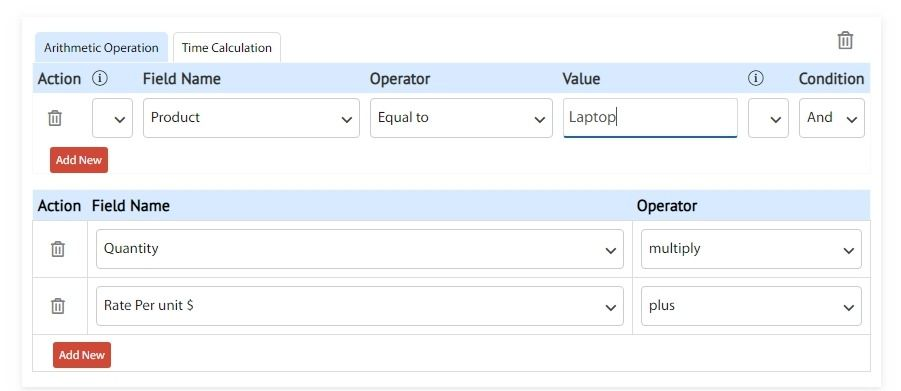Cflow provides an Arithmetic Operation feature to perform simple math operations and automatically populate the result in a field. You can perform arithmetic operations if the data type is decimal and integer.

In Arithmetic Operation, you can specify how a field value is calculated and displayed using math operations (plus, minus, multiply, divide and remainder). You may perform math operations between multiple fields and constant values. You may also create conditional logic to calculate different field values based on different rules.

For example, imagine a situation where a Total Price field needs to be dynamically determined by multiplying the Quantity and Rate per unit. Let’s also say that this needs to be done only if the Product is ‘Laptop’. In such a case, you will configure the Arithmetic Operation section as shown below: